## Thursday, August 9, 2007

### Irodov Problem 1.71To a person in an accelerating elevator thats accelerating upwards at rate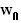then the person of mass m will feel as if the gravitational is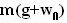. The extra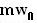is some times referred to also as a pseudo force that a person in an accelerated frame experiences. In fact this also lead to Einstein's famous principle of equivalence. So we can solve the problem in the accelerated frame of reference of the elevator as if the effective gravitational constant were. However, here we shall solve with respect to a stationary frame.

Let T be the tension in the pulley string, F be the force between the pulley and the elevator car. Further let us assume that the acceleration of massrelative to the elevator car be w directed towards the elevator floor. Massthen accelerates at a rate w towards the elevator ceiling relative to the elevator car. The net accelerations ofandrelative to a stationary observer are thus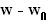andtowards the elevator floor respectively.

Forces on mass: There are two forces acting on this mass i) gravity and ii) tension in the pulley string as shown in the figure. Thus, its dynamics are given by,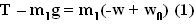Forces on mass: There are two forces acting on this mass i) gravity and ii) tension in the string as shown in the figure. Thus, its dynamics are given by,We can now solve of w from (1) and (2) by subtracting the two equations as,From (3) now we can determine the acceleration ofrelative to the shaft in the direction towards the floor (stationary observer) as,Forces acting on the Pulley:
There are three force acting on the pulley i) tension T in the part of the string connecting mass, ii) tension T in the part of the string connecting mass, and iii) the force the elevator exerts on the pulley F. The pulley is accelerating with an acceleration ofupwards but the pulley has no mass. Thus, we have,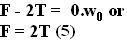Tension T can be calculated from (1) and (4), as,From (6) the force exerted on the elevator on the pulley F isAnonymous said...

dear mr. krishna kant..
you seem to be very elder to me for im passing the age u have described..
the fact of the mattter is that i did the same solution to the problem using psuedo forces n without it but reached the same answer as you did.as you might know our ansers dont match with what the author has provided(ans being (m1-m2)(g-W)/m1+m2..
this author's ans has somehow been also reached to by mr.ap singh in his book and a russian website..
i would like to know if there is something we are missing and thus our ans nt matching...or if you are confident that irodov made a mistake...
my email is dig_v_j@yahoo.com
thanx...

Krishna Kant Chintalapudi said...

dear Dig_v_j,

Irodov is a great man but nevertheless he is a human and can sometimes make a mistake. In this problem I believe he has made an inadvertent mistake. If he had changed the direction of the acceleration of the elevator (down instead of up) the answers would match.

To verify consider wo = g, according to Irodovs answer we would get a 0 in all the parts.
This would be true if the elevator were accelerating downwards (ie experiencing a free fall) at a rate 'g' not upwards. Under free fall relative to the elevator there would be no gravity.

So yes you are right and Irodov is wrong in this answer. If someone has matched Irodov's answer, they are wrong too. I know its hard to believe in yourself when Dr. Irodov says something but I am sure it was a silly mistake on his part. We should excuse the great man :-) for this.

-krishna kantAnonymous said...

dear mr.krishna,.
as a matter of fact,,i have discovered that in fact Irodov was not wrong in his answer..
the answer actually has vector signs involved which we misinterpretted.
the answer (m2-m1)(g-w)/(m1+m2) is correct because the letters g and w have been wriiten in bold letters i.e. they are vectors.so since a and g have opposite signs(considering directions)when we actually do vector g minus vector w it comes to be g-(-w)=g+w which is our answer.so Irodov and us are both correct ,he being more correct as he took the vectors in considerations.
thank you,
digvijay,class XI

Krishna Kant Chintalapudi said...

Dear digvijay,

Yes you are right, in vectorial notation the Irodov solution is indeed right.

I will correct the solution in the next few days. Thanks for pointing this out.

-krishnaAnonymous said...

Sir I believe your soluttion is right
student
IIT Bombay